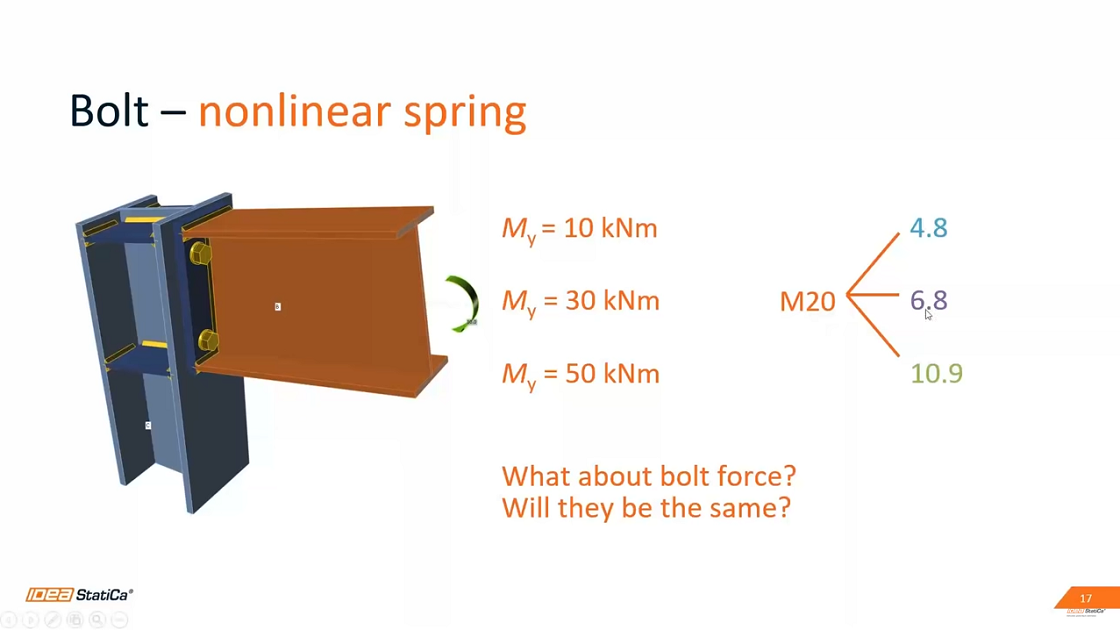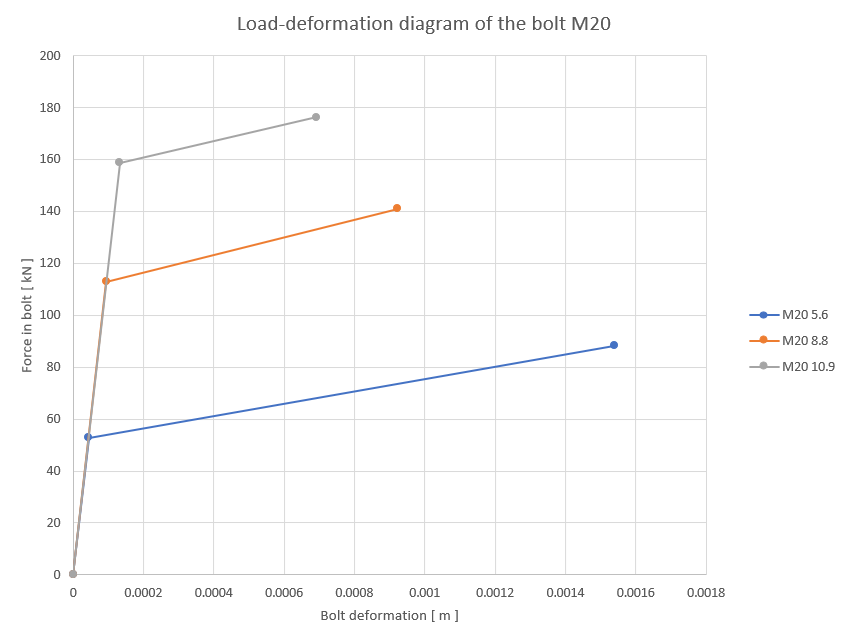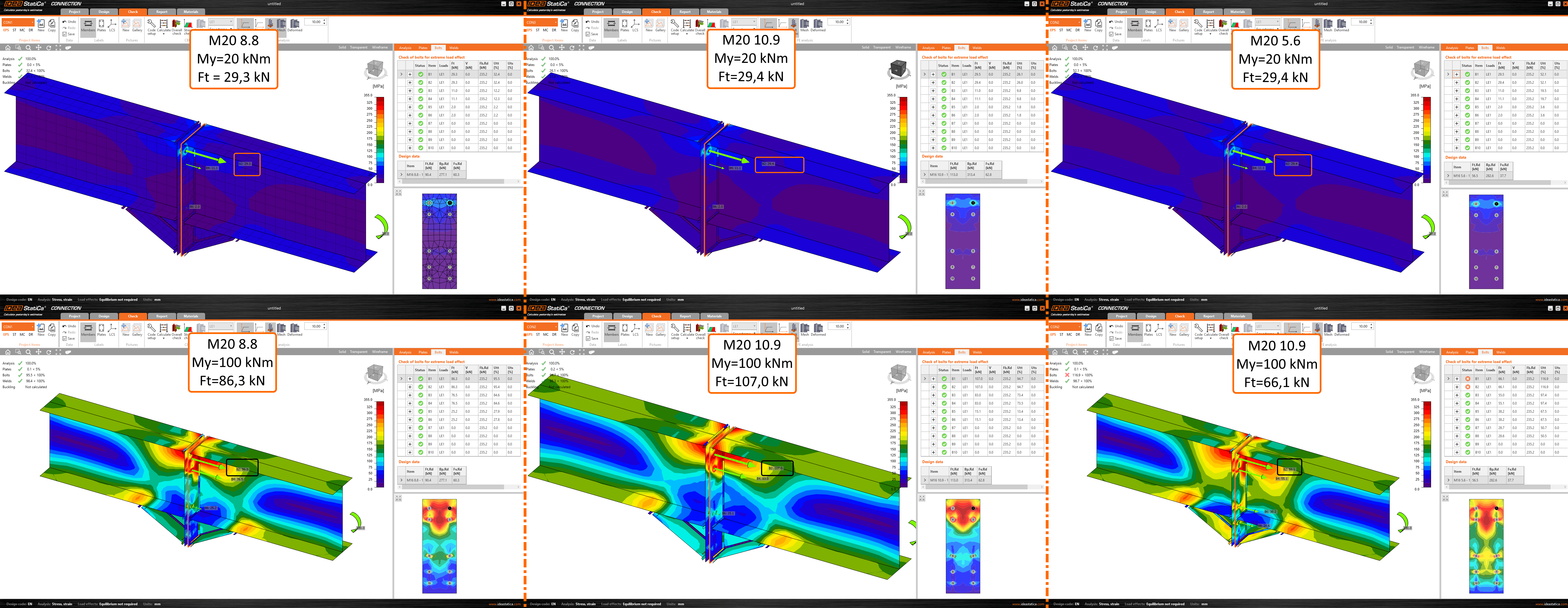### Choose language# Different tension forces in the bolts

$$Why are the tension forces in bolts sometimes different for each bolt grade? Each bolt grade has a different working diagram and different value of yield strength. In the CBFEM model of a joint used in IDEA StatiCa applications, the deformation of plates and other parts is calculated as a part of the complex analysis. Due to the deformation, there are extra loads introduced to the bolts known as prying forces. Depending on the applied load effects and the prying forces, the resulting force in bolt may variate a lot for different bolt grades.On the model of and endplate connecting two beams loaded by a bending moment, the comparison of three different bolt grades (8.8, 10.9 and 5.6) of the same bolt size M20 shows that for low applied force the bolt forces result in the same values while raising the applied force results in variation in the bolt forces.For more information see the theoretical background and the video below.$$